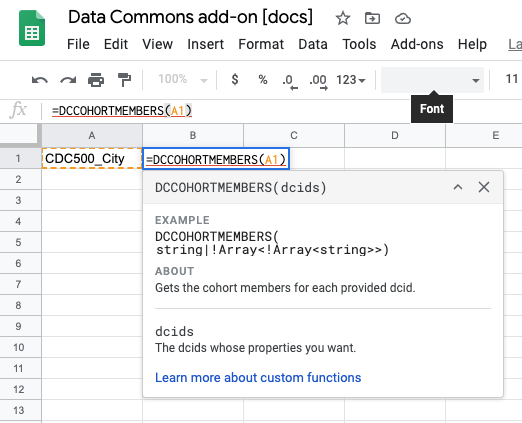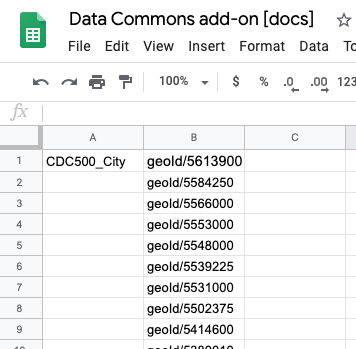# Get the values of a given property for a node.

## `=DCCOHORTMEMBERS(dcids)`

Given a cohort or a row of cohort DCIDs, get the members of each cohort.

Arguments

• `dcids` - cohort DCIDs to get the members of

Returns

The DCIDs of the cohort members. For a single DCID, the result is a column of members. For a row of DCIDs, the result is a matrix with each column being the members of the DCID at the column’s index. For a column of DCIDs, the result is a matrix with each row being the members of the DCID at the row’s index.

## Examples

In this example, we find the members of the CDC500_City cohort.

### Input### Output (truncated)